Select Page

## Using Variables to Draw: Squiral

### Homework 5: Drawing a Squiral

The squiral is a cross between a square and a spiral.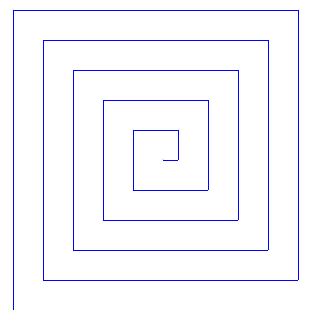It looks like you start off drawing a square, but then you end up with something like a spiral. In this homework, we’re going to write a script that draws this squiral.

Which is the longest side of the squiral? Click on the side to move the green dot. When the dot is in the correct location (anywhere on the side), press Check.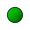Which is the shortest side of the squiral? Click on the side to move the green dot. When the dot is in the correct location (anywhere on the side), press Check.Based on where the sprite is, was the shortest side or the longest side drawn first?To draw a squiral, it’s a good idea to start by drawing a square. It’s probably easier to go from a square to a squiral than from a spiral to a squiral. (Think about how you might change a spiral to look like a squiral. It’s not that easy!)

We can start off with a square with sides that are 30 steps long. (This length makes it so we can see the sides and the sprite at the same time! You can start with sides that are shorter or longer.)We can write a shorter version of this script using a repeat block.

What is the smallest number of blocks you would need to make this script?

But how do we go from this square script to a squiral? We can figure this out by looking at a small piece of the squiral first. Then we can figure out how to turn a square into a squiral. Let’s look at the “square” in the middle of the squiral. The sides are labeled 1, 2, 3, and 4 to make it easier to talk about them.In the first question, we saw that the shortest side (side 1) gets drawn first and the longest side (side 4) gets drawn last. This means that as the sprite draws the squiral, the sides get longer.

Fill in the blank:

If the sprite drew another side (side 5) of the squiral, the new side would be ____________________ side 4.

Side 5 would be angled _______________.

Side 5 would be located at the _______________ of the squiral.

Let’s look at our script for the square again:Which lines of the script draw side 2? Check all that apply.

Which lines of the script draw side 3? Check all that apply.

Which lines of the script draw side 4? Check all that apply.

Now that we’ve figured out which parts of the script draw each side, we can change it to draw a squiral.

Which lines of the script need to change so that side 2 is longer than side 1, side 3 is longer than side 2, and side 4 is longer than side 3? Check all that apply.

Hint: Should we change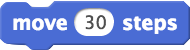or?

Once we change the parts that you circled, we’ll probably end up with something that looks like this:We can see that the length of each side goes up by 10 (you don’t have to change by 10 though! Is it better to have the length of each side go up by the same or by a different amount?).

If we include ablock at the end of this script, we can see our squiral much better: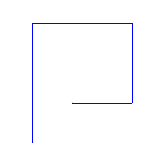If we want to show the sprite again, we can remove theblock and add ablock. We will see the sprite again when we run the script with this block.

Now we know how to make a 4-sided squiral. But what do we need to do if we want to make a 20-sided squiral?

We could just take our script for the 4-sided squiral and add more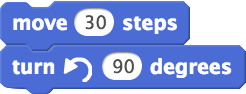blocks. But we would need 16 more of these two blocks to make a 20-sided squiral! That’s a lot!

We would also end up doing a lot of math because if we just addblocks, we have to figure out the length of each side by hand to change all of theblocks. Is there a way to change the length of each side without having to figure it out by hand? Let’s try to figure this for the 4-sided squiral.

We know that the sides of the squiral get longer as the sprite draws them. Side 2 is longer than side 1, side 3 is longer than side 2, and side 4 is longer than side 3.If we define length to be the length of side 1 (length = length of side 1), then the length of side 2 is length +

Hint: Side 2 is longer than side 1 by how many steps?

If we define length to be the length of side 2 (length = length of side 2), then the length of side 3 is length +

If we define length to be the length of side 3 (length = length of side 3), then the length of side 4 is length +

This may seem a bit weird, but we see that the length changes by 10 every time. (For example, the length of side 4 = length of side 3 + 10.) So maybe we can make a variable calledand every time we draw a new side, we can. Then, when we move the sprite, we caninstead of.

What should the value ofbe when we start drawing the squiral so it is the same size as our squiral without variables?

Now we can fit this into our script for the 4-sided squiral:This looks exactly like what we drew before!Just like the script for drawing a square, we can write a shorter version of this script using repeat.

What is the smallest number of blocks you would need to make this script?

Hint: Think about how this script is similar to or different from the script for the square. Look back to question 2!

We should end up with a script that looks like this:What part of this script would you change to draw a 20-sided squiral?This code will draw: